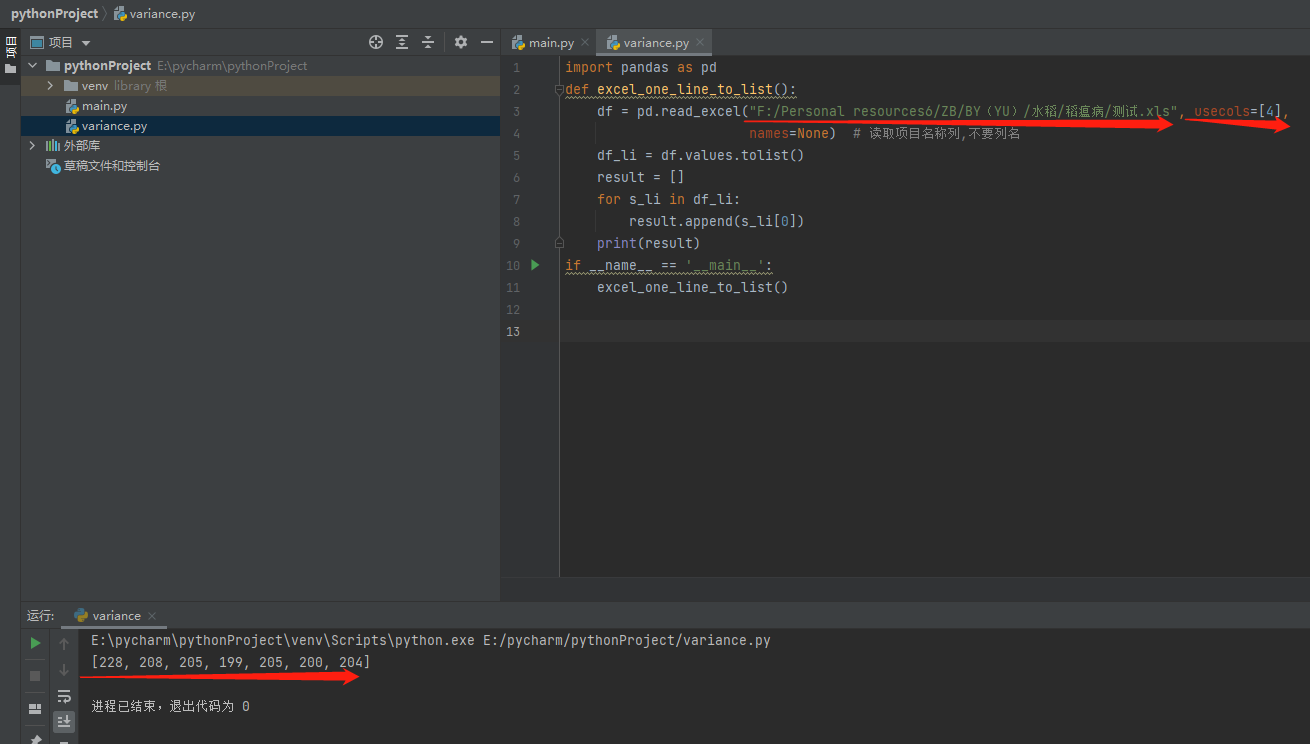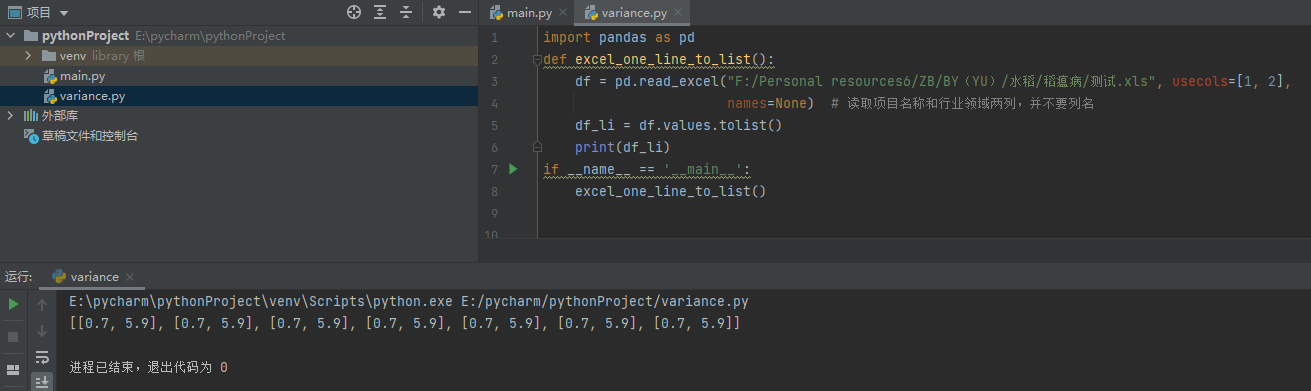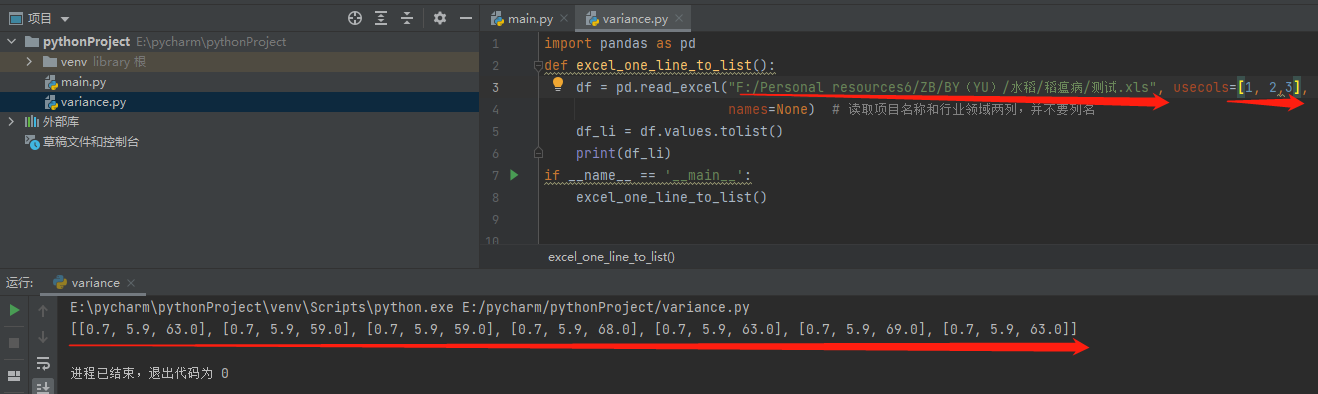• python读取excel某列数据

万次阅读 多人点赞 2020-12-19 16:16:26
文章目录python读取excel列数据二、将读取的数据变为浮点数 python读取excel列数据 import xlrd worksheet = xlrd.open_workbook('E:\\Crawl\\000002.xls') sheet_names= worksheet.sheet_names() ...

一、python读取excel某列数据

import xlrd

worksheet = xlrd.open_workbook('E:\\Crawl\\000002.xls')
sheet_names= worksheet.sheet_names()
print(sheet_names)
for sheet_name in sheet_names:
sheet = worksheet.sheet_by_name(sheet_name)
rows = sheet.nrows # 获取行数
cols = sheet.ncols # 获取列数，尽管没用到
all_content = []

cols = sheet.col_values(3) # 获取第二列内容， 数据格式为此数据的原有格式（原：字符串，读取：字符串；  原：浮点数， 读取：浮点数）
print(cols)
print(cols)
print(type(cols))    #查看数据类型

输出结果为：

['Sheet1']
['', '', '-72.20', '248.84', '-32.67', '156.93', '-49.58', '59.36', '']
248.84
<class 'str'>

二、将读取的数据变为浮点数

import xlrd

worksheet = xlrd.open_workbook('E:\\Crawl\\000002.xls')
sheet_names= worksheet.sheet_names()
print(sheet_names)
for sheet_name in sheet_names:
sheet = worksheet.sheet_by_name(sheet_name)
rows = sheet.nrows # 获取行数
cols = sheet.ncols # 获取列数，尽管没用到
all_content = []

for i in range(rows) :
cell = sheet.cell_value(i, 3) # 取第二列数据
try:
cell = float(cell) # 转换为浮点数
all_content.append(cell)
except ValueError:
pass
print(all_content)
print(all_content)
print(type(all_content))

结果为：

['Sheet1']
[-72.2, 248.84, -32.67, 156.93, -33.53, 64.06, -47.0, 117.33, -31.6, 62.56, -33.79, 30.63, -59.65, 53.36, -57.73, 34.15, -60.0, 161.36, -60.0, 41.3, -50.77, 375.31, -43.66, 167.79, -57.47, 62.4, -53.31, 98.65, -37.41, 68.59, -42.04, 54.28, -58.9, 64.21, -51.92, 36.0, -49.02, 630.77, -35.75, 160.82, -51.98, 31.93, -80.17, 198.75, -35.77, 34.31, -46.24, 84.36, -46.82, 321.93, -33.41, 120.85, -49.58, 59.36]
156.93
<class 'float'>

展开全文python
• Excel表格是我们处理数据的工具，在Python编程中，也有对excel表格的操作。...python读取excel列数据importxlrdworksheet=xlrd.open_workbook('E:\\Crawl\\000002.xls')sheet_names=worksheet....Excel表格是我们处理数据的工具，在Python编程中，也有对excel表格的操作。本文主要向大家介绍python中读取excel某列数据的代码，并演示将读取的数据变为浮点数的过程。

一、python读取excel某列数据import xlrd

worksheet = xlrd.open_workbook('E:\\Crawl\\000002.xls')

sheet_names= worksheet.sheet_names()

print(sheet_names)

for sheet_name in sheet_names:

sheet = worksheet.sheet_by_name(sheet_name)

rows = sheet.nrows # 获取行数

cols = sheet.ncols # 获取列数，尽管没用到

all_content = []

cols = sheet.col_values(3) # 获取第二列内容， 数据格式为此数据的原有格式(原：字符串，读取：字符串；  原：浮点数， 读取：浮点数)

print(cols)

print(cols)

print(type(cols))    #查看数据类型

输出结果为['Sheet1']

['', '', '-72.20', '248.84', '-32.67', '156.93', '-49.58', '59.36', '']

248.84

二、将读取的数据变为浮点数import xlrd

worksheet = xlrd.open_workbook('E:\\Crawl\\000002.xls')

sheet_names= worksheet.sheet_names()

print(sheet_names)

for sheet_name in sheet_names:

sheet = worksheet.sheet_by_name(sheet_name)

rows = sheet.nrows # 获取行数

cols = sheet.ncols # 获取列数，尽管没用到

all_content = []

for i in range(rows) :

cell = sheet.cell_value(i, 3) # 取第二列数据

try:

cell = float(cell) # 转换为浮点数

all_content.append(cell)

except ValueError:

pass

print(all_content)

print(all_content)

print(type(all_content))

以上就是python中读取excel某列数据的代码，以及演示将读取的数据变为浮点数的过程。大家可以直接套用使用哦~希望能对你的python学习有所帮助。

展开全文• 如下所示：#encoding=utf-8import xlrdfrom xlwt import *#------------------读数据---------------------------------fileName="C:\\Users\\st\\Desktop\\test\\20170221131701.xlsx"bk=xlrd.open_workbook...

如下所示：

#encoding=utf-8

import xlrd

from xlwt import *

#------------------读数据---------------------------------

fileName="C:\\Users\\st\\Desktop\\test\\20170221131701.xlsx"

bk=xlrd.open_workbook(fileName)

shxrange=range(bk.nsheets)

try:

sh=bk.sheet_by_name("Sheet1")

except:

print "代码出错"

nrows=sh.nrows #获取行数

book = Workbook(encoding='utf-8')

for i in range(1,nrows):

row_data=sh.row_values(i)

#获取第i行第3列数据

#sh.cell_value(i,3)

#---------写出文件到excel--------

print "-----正在写入 "+str(i)+" 行"

sheet.write(i,1, label = sh.cell_value(i,3)) #向第1行第1列写入获取到的值

sheet.write(i,2, label = sh.cell_value(i,5)) #向第1行第2列写入获取到的值

book.save("C:\\Users\\st\\Desktop\\test\\demo1.xls")

以上这篇python读取excel指定列数据并写入到新的excel方法就是小编分享给大家的全部内容了，希望能给大家一个参考，也希望大家多多支持萬仟网。

如您对本文有疑问或者有任何想说的，请点击进行留言回复，万千网友为您解惑！

展开全文• Pandas 是 Python 语言的个扩展程序库，用于数据分析。 Pandas 可以从各种文件格式比如 CSV、JSON、SQL、Microsoft Excel 导入数据。 Pandas 的主要数据结构是 Series （数据）与 DataFrame（二维数据），这两...

Pandas 是 Python 语言的一个扩展程序库，用于数据分析。
Pandas 可以从各种文件格式比如 CSV、JSON、SQL、Microsoft Excel 导入数据。
Pandas 的主要数据结构是 Series （一维数据）与 DataFrame（二维数据），这两种数据结构足以处理金融、统计、社会科学、工程等领域里的大多数典型用例。
python操作excel主要用到xlrd和xlwt这两个库，即xlrd是读excel，xlwt是写excel的库。这里需要用到的是"xlrd"。
（一）Python读取excel中的一列并转为数组
只需要修改一个文件路径即可读取，usecols后面的数字表示第几列

import pandas as pd
def excel_one_line_to_list():
names=None)  # 读取项目名称列,不要列名
df_li = df.values.tolist()
result = []
for s_li in df_li:
result.append(s_li)
print(result)
if __name__ == '__main__':
excel_one_line_to_list()（二）Python读取excel中的多列并转为数组
只需要修改一个文件路径即可读取，usecols后面的第一个数字表示第一列，第二个表示第二列

import pandas as pd
def excel_one_line_to_list():
df = pd.read_excel("F:/Personal resources6/ZB/BY（YU）/水稻/稻瘟病/测试.xls", usecols=[1, 2],
names=None)  # 读取项目名称和行业领域两列，并不要列名
df_li = df.values.tolist()
print(df_li)
if __name__ == '__main__':
excel_one_line_to_list()展开全文python
• 我的是为了生成个数组，将matlab生成的数据导到单片机中'''import pandas as pddef excel_one_line_to_list():df = pd.read_excel("C:/Users/lenovo/Desktop/11.xlsx", usecols=,names=None) # 读取项目名称,...
• python读取excel数据

千次阅读 2020-12-28 20:12:57
by_name(sheetname) '''获取第数据，即表头''' self.keys = self.data.row_values(0) '''获取总行数''' self.rowsNum = self.data.nrows '''获取总数''' self.colsNum = self.data.ncols def dict_data(self):...
• 读取一列保存为list，项目名称为例：import pandas as pddef excel_one_line_to_list():df = pd.read_excel("/Users/Devintern/Documents/pachong/ML_flow/pachong/qmp/files/投资事件导出20190303.xlsx", usecols=...
• I am using python xlwings to read a column of data in Excel 2013. Column A is populated with numbers. To import this column into a python list py_list, I have the following code;import xlwings as xwwb...
• 用Python读取excel中的数据

千次阅读 2021-04-26 20:40:53
python中要想处理excel，必须用到第三方模块xlrd，所以windows 我安装方法是cmd中命令：E:\ANZHUANG\Python\Scripts>easy_install xlrd (路径必须在python的scripts下和必须安装easy_install)#coding=utf-8...
• Worksheet 对象的 rows 属性和 columns 属性得到的是 Generator 对象，不能用中括号取索引。...补充：python之表格数据读取python 操作excel主要用到xlrd,xlwt这两个库，xlrd,是读取excel表，xlwt是写入表格...
• import xlrdimport datetimefile=u"伏特加.xls"#注意读中文文件名稍微处理一下data=xlrd.open_workbook(file)table = data.sheet_by_index(0)#按照索引读Excel文件colContent=table.col_values(1)#读某一列，...
• 利用python处理excel数据：将其读取出来，并且最终实现数据相乘，打印出相乘的结果，其他的运算如加减都可以类比得出 实质在于，将其转化为个列表，接着进行赋值 以下的代码很简单，相当于个模板，在数据...python excel
• df = pd.read_excel('2021MCMProblemC_DataSet.xlsx') # select the right data reports = df.loc[(df['Lab Status'] == 'Positive ID'), ['Detection Date', 'Latitude', 'Longitude']] ndata = np.array(reports)python
• #读取excel表格数据 df = pd.read_excel( r"E:\a研究生学习\项目\数据\h2.xlsx") print(df) #输出表格 print(df.dtypes) #显示表格的数据类型 #输出 m1 m2 m3 m4 0 6082 80 2 3 1 2132 80 4 3 2 9857 80 .python pycharm
• 1.在Windows命令行中安装第三方模块xlrd，先切到python安装目录(Python34)，...新建excel表，然后保存，造数据3.编写python脚本#utf-8import xlrd #导入第三方模块xlrdexcel = xlrd.open_workbook('C:\\表格.xl...
• python读取excel要看你的excel表文件的存储格式，一般需要先pip install xlrd安装xlrd库，如果你的表格拓展名为xlsx格式，解析表格应该会报Excel xlsx file； not supported的错误，这是因为默认安装的xlrd库是最新...python 开发语言
• 最近在工作之余学习了Python，在学到Python操作excel文件的时候，基于我们项目上接口自动化获取参数的方法，练习了一下获取excel文件的某一列的接口值，并把它保存在一个列表上，以此作为以后复习的记录。...
• ,要读取数据的格式：二,数据读取部分：b站视频参考：https://www.bilibili.com/video/BV14C4y1W7Nj?t=148# 1930workbook=xlrd.open_workbook('1930.xlsx')sheet= workbook.sheet_by_index(0)A1=[]B1=[]# sheet....
• 1.读取Excel 2.获取内容后并传递给相应的参数 import requests import xlrd import time def read_excel(): workbook = xlrd.open_workbook('data.xlsx') table = workbook.sheet_by_name(u'Sheet1') n...
• 通过Python读取Excel数据并打印 这段时间了解了下同事们做报表，很多还在手动操作。很多机械性的处理操作，其实就可以通过Python写个脚本直接跑。然后我就来了解一下，到处都在宣传的Python做报表的Skill。 个人...python excel 数据分析
• 问题描述：已有Excel文件，其中包含5列数据，要求在第3列前插入一列数据，保存为新文件。参考代码：运行结果：--------董付国老师Python系列教材--------1）《Pytho...excel 数据分析 大数据 python csv
• 我遇到了个问题的过程中写数据Excel。输入的数据写在for循环,这是写在多次(为每个for循环次,和几十个数据一次)。但当数据写在第二个for循环,第次写的数据会被覆盖,即数据写在下次将覆盖数据之前写的。代码...
• python逐行读取excel

千次阅读 2021-01-11 20:24:31
python读取excel文件，将每行都保存为个列表。比如：文件是a,b,d f,e,g u,w,o 想要变成list1=[a,b,c] list2=[f,e,g] lpython读写excel文件要用到两个库：xlrd和xlwt，首先下载安装这两个库。#读取Excel import ...
• 今日给大家分享Python读取Excel表格，同时采用表格中的数值画图柱状图和折线图，这里只需要几行代码便可以实。首先我们需要安装个Excel操作的库xlrd，这个很简单，在安装Python后直接在DOS命令下输入pip ...
• 错误处理 DB api中定义了一些数据库操作的错误及异常，下表出了这些错误和异常:异常描述 Warning当有严重警告时触发，例如插入数据是被截断等等。必须是 StandardError 的子类。 Error警告以外所有其他错误类。......

python读取excel一列数据python 订阅